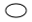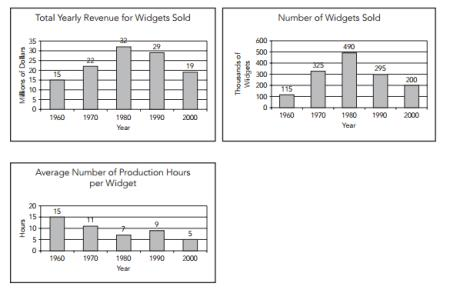# GRE Quantitative Reasoning

Quantitative Reasoning in GRE General Test covers arithmetic, algebra, geometry, and data analysis, and its question types are usually quantitative comparison, numeric entry, word problem, and data interpretation. Here we offer hundreds of GRE math exercises grouped by content and question type to practice online.

 Select Exercise Lists

By Question Type

By Content

 Overview and Practice of GRE Quantitative Reasoning
1 Overview
GRE general test includes a math-related section: quantitative reasoning. It evaluates the ability to reason quantitatively and to address problems with quantitative methods, or say necessary mathematical skills.

The questions are in two sorts:
• Pure mathematical problems: answer by math knowledge only
• Word problems: answer by modeling problems mathematically
The mathematical symbols, terminology, and conventions in the Quantitative Reasoning worksheets are understandable at the high school level. Besides, there are some other assumptions listed in the Quantitative Reasoning section directions:
• All numbers used are real numbers.
• All figures are in a plane unless otherwise indicated.
• Geometric figures are not necessarily drawn to scale.
• Coordinate systems are drawn to scale
You can use a calculator in the test, but you cannot use your own calculator. In the computer-based GRE test, the calculator is provided on-screen; as for paper-delivered test, the test center will offer a basic calculator. You may get latest and official information of GRE Quantitative Reasoning from Quantitative Reasoning Measure.

2 GRE Quantitative Reasoning
GRE Quantitative Reasoning isn't real math test. It doesn't test all high school math skills; for example, it does not include trigonometry, calculus, or other higher-level mathematics. Instead, it just covers four primary math parts: arithmetic, algebra, geometry, and data analysis.

Arithmetic Topics include properties and types of integers, arithmetic operations, exponents and roots, and concepts of estimation, percent, absolute value, the number line, and decimal representation.

Example:

 n is an even integer Quantity A Quantity B The remainder when n is divided by 3 The remainder when n is divided by 5Quantity A is greater.Quantity B is greater.The two quantities are equal.The relationship cannot be determined without further information.

Algebra Topics include operations with exponents; algebraic expressions; equations and inequalities; linear and quadratic equations and inequalities; and coordinate geometry, including graphs of functions, intercepts and slopes of lines.

Example:

 Quantity A Quantity B x4 / 4 (x / 4)4Quantity A is greater.Quantity B is greater.The two quantities are equal.The relationship cannot be determined without further information.

Geometry Topics include parallel and perpendicular lines, circles, triangles, quadrilaterals, other polygons, three-dimensional figures, area, perimeter, volume, and angle measurement in degrees. (Not need to construct proofs of geometry.)

Example:

 10mm = 1 cm Quantity A Quantity B The area of a square with sides of one centimeter 10 times the area of a square with sides of 1mmQuantity A is greater.Quantity B is greater.The two quantities are equal.The relationship cannot be determined without further information.

Data Analysis Topics include basic descriptive statistics; interpretation of data in tables and graphs; elementary probability; conditional probability; random variables and probability distributions, including normal distributions; and counting methods, such as combinations and permutations.

Example:
10.

 Quantity A Quantity B The sum of the even numbers from 2 to 100 inclusive 52 x 50Quantity A is greater.Quantity B is greater.The two quantities are equal.The relationship cannot be determined without further information.

3 GRE Quantitative Reasoning Question Type
GRE Quantitative Reasoning has four common types of questions. A question may be promoted in two ways: either independently as a discrete question or as part of a set of questions, the latter case is usually in Data Interpretation whose all questions are based on the same data source, like tables or graphs.

Quantitative Comparison is the primary type in GRE quantitative reasoning and usually ask you select one answer choice from multiple options.

Example:

 A jar of 90 marbles contains marbles of three different solid colors: red, blue and green. More than half the marbles are either red or blue. Quantity A Quantity B The number of blue marbles in the jar Twice the number of green marbles in the jarQuantity A is greater.Quantity B is greater.The two quantities are equal.The relationship cannot be determined without further information.

Numeric Entry needs you calculate the answer; some Words Problem question also needs you give the numeric answer after modeling.

Example:
OmniCorp's call center operates 24 hours a day and receives an average of 40 customer-service calls per hour. 78 percent of all calls are received during the night shift. How many customer-service calls are received during this shift, on average?

Word Problem emphasizes to translate the problem to the mathematical model, you need to calculate the answer, select one or more choices from multiple options.

Example:
If 13 + x3 + 83 = 93, then x = ?-10169

Data Interpretation usually has a set of questions based on the same data source; the problems are diversity, like calculating the answer, selecting one or more choices from multiple options.

Example:1. In 1990, the total revenue for widgets sold was about how many times as great as the number of widgets sold?3106598120
2. . In 1970, if the cost to produce one widget was \$0.07, what would have been the total cost to produce the number of widgets sold during that year?\$1,540\$14,000\$20,650\$22,750\$34,300
3. . The percent decrease in average number of production hours per widget from 1990 to 2000 was approximately44%36%34%32%27%
4. . In how many of the years shown was the number of widgets sold at least three times the number of widgets sold in 1960?FourThreeTwoOneNone
5. . Which of the following statements can be inferred from the data?
I. The greatest increase in total yearly revenue over any 10-year period shown was \$7 million.
II. In each of the 10-year periods shown in which total yearly revenue increased, the average number of production hours per widget decreased.
III. From 1980 to 1990, fewer widgets were sold because each widget took longer to produce.I onlyII onlyIII onlyI and IIIII and III